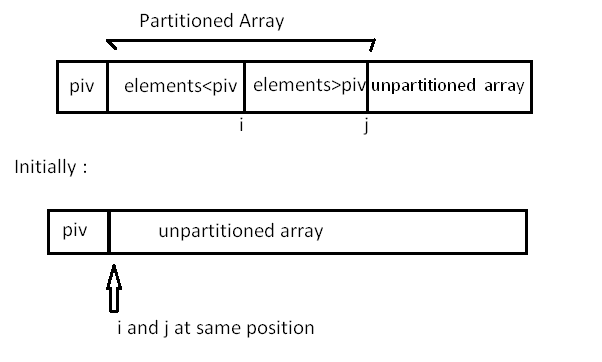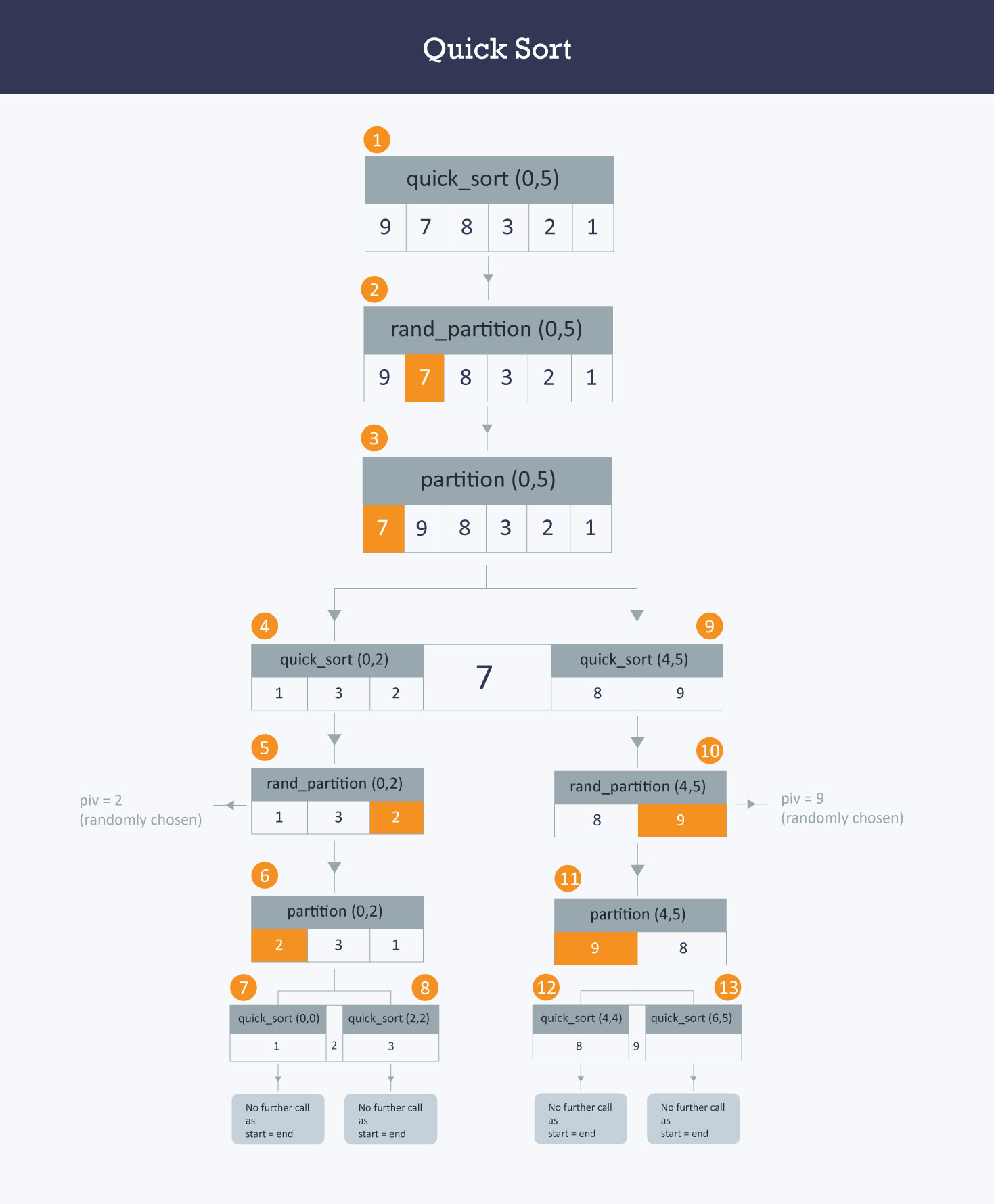Algorithms
Topics:
Quick Sort
• Searching
• Sorting
• Greedy Algorithms
• Graphs
• String Algorithms
• Dynamic Programming

# Quick Sort

Quick sort is based on the divide-and-conquer approach based on the idea of choosing one element as a pivot element and partitioning the array around it such that: Left side of pivot contains all the elements that are less than the pivot element Right side contains all elements greater than the pivot

It reduces the space complexity and removes the use of the auxiliary array that is used in merge sort. Selecting a random pivot in an array results in an improved time complexity in most of the cases.

Implementation :

Select the first element of array as the pivot element First, we will see how the partition of the array takes place around the pivot.In the implementation below, the following components have been used: Here, $A[]$ = array whose elements are to be sorted

$start$: Leftmost position of the array

$end$: Rightmost position of the array

$i$ : Boundary between the elements that are less than pivot and those greater than pivot

$j$ : Boundary between the partitioned and unpartitioned part of array

$piv$: Pivot element

int partition ( int A[],int start ,int end) {
int i = start + 1;
int piv = A[start] ;            //make the first element as pivot element.
for(int j =start + 1; j <= end ; j++ )  {
/*rearrange the array by putting elements which are less than pivot
on one side and which are greater that on other. */

if ( A[ j ] < piv) {
swap (A[ i ],A [ j ]);
i += 1;
}
}
swap ( A[ start ] ,A[ i-1 ] ) ;  //put the pivot element in its proper place.
return i-1;                      //return the position of the pivot
}


Now, let us see the recursive function Quick_sort :

void quick_sort ( int A[ ] ,int start , int end ) {
if( start < end ) {
//stores the position of pivot element
int piv_pos = partition (A,start , end ) ;
quick_sort (A,start , piv_pos -1);    //sorts the left side of pivot.
quick_sort ( A,piv_pos +1 , end) ; //sorts the right side of pivot.
}
}Here we find the proper position of the pivot element by rearranging the array using partition function. Then we divide the array into two halves left side of the pivot (elements less than pivot element) and right side of the pivot (elements greater than pivot element) and apply the same step recursively.

Example: You have an array $A=[9,7,8,3,2,1]$ Observe in the diagram below, that the $randpartition()$ function chooses pivot randomly as $7$ and then swaps it with the first element of the array and then the $partition()$ function call takes place, which divides the array into two halves. The first half has elements less than $7$ and the other half has elements greater than $7$.
For elements less than $7$, in $5^{th}$ call, $randpartition()$ function chooses $2$ as pivot element randomly and then swap it with first element and call to the $partition()$ function takes place. After the $7{th}$ and $8{th}$ call, no further calls can take place as only one element left in both the calls. Similarly, you can observe the order of calls for the elements greater than $7$.

Let’s see the randomized version of the partition function :
int rand_partition ( int A[ ] , int start , int end ) {
//chooses position of pivot randomly by using rand() function .
int random = start + rand( )%(end-start +1 ) ;

swap ( A[random] , A[start]) ;        //swap pivot with 1st element.
return partition(A,start ,end) ;       //call the above partition function
}


Use $randpartition()$ instead of $partition()$ function in $quicksort()$ function to reduce the time complexity of this algorithm.

Complexity The worst case time complexity of this algorithm is $O(N^2)$ , but as this is randomized algorithm, its time complexity fluctuates between $O(N^2)$ and $O(NlogN)$ and mostly it comes out to be $O(NlogN)$

Contributed by: Anand Jaisingh

?